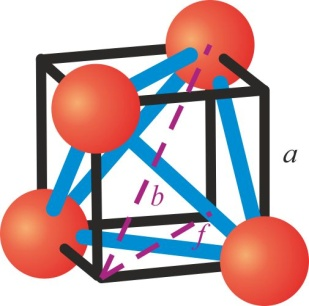# Problem: A tetrahedral site in a close-packed lattice is formed by four spheres at the corners of a regular tetrahedron. This is equivalent to placing the spheres at alternate corners of a cube. In such a close-packed arrangement the spheres are in contact and if the spheres have a radius r, the diagonal of the face of the cube is 2r. The tetrahedral hole is inside the middle of the cube.Find the length of the body diagonal of this cube.

###### FREE Expert Solution

We are asked to find the length of the body diagonal of the given cube.Recall:

The length of a body diagonal of a cube is:

$\overline{){\mathbf{d}}{\mathbf{=}}\sqrt{\mathbf{3}}{\mathbf{a}}}$

The length of a face diagonal of a cube is:

$\overline{){\mathbf{d}}{\mathbf{=}}\sqrt{\mathbf{2}}{\mathbf{a}}}$

where a is the edge length of a cube

90% (95 ratings)###### Problem Details

A tetrahedral site in a close-packed lattice is formed by four spheres at the corners of a regular tetrahedron. This is equivalent to placing the spheres at alternate corners of a cube. In such a close-packed arrangement the spheres are in contact and if the spheres have a radius r, the diagonal of the face of the cube is 2r. The tetrahedral hole is inside the middle of the cube.

Find the length of the body diagonal of this cube.